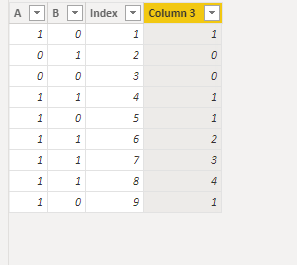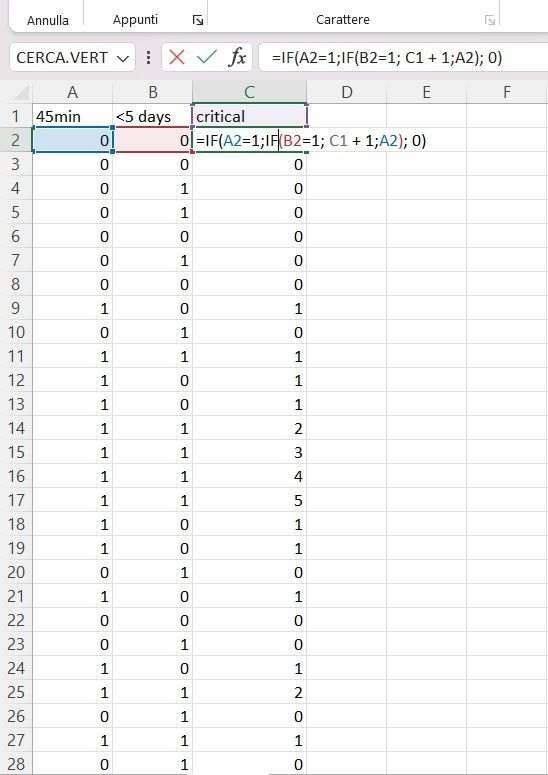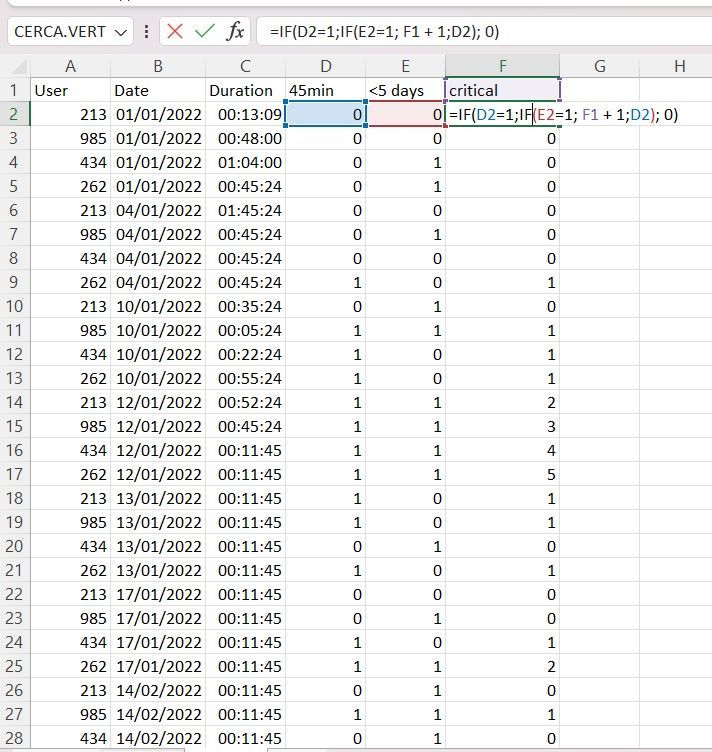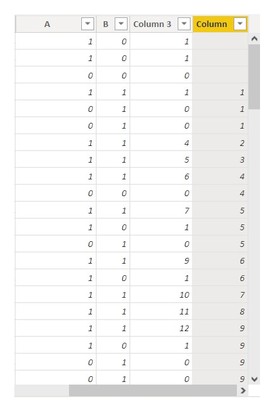cancel
Showing results for
Did you mean:

Fabric is Generally Available. Browse Fabric Presentations. Work towards your Fabric certification with the Cloud Skills Challenge.Frequent Visitor

## Need help with my code - incremental calculated column DAX

Hi everyone,

I need help fixing my code for an incremental column based on 2 conditions.

Here it is:It is not working properly because you can see in row 16 I don't want to be shown a 3, but a 2. You see it is happening because the column is based on a calculation that gives back the difference between number of rows so I need to add one condition to my code in order to fix this. The problem is, I can't figure out how.

This is the code:

`Column 3 = VAR c = 'Tot MD_TEST'[Data]VAR pre =    CALCULATE (        MAX ( 'Tot MD_TEST'[Data] ),        FILTER ( 'Tot MD_TEST',         'Tot MD_TEST'[Data] < c         && 'Tot MD_TEST'[A] = 0        && 'Tot MD_TEST'[Name] = EARLIER('Tot MD_TEST'[Name])    )    )VAR data_prec =    CALCULATE(           MAX('Tot MD_TEST'[Data]),           FILTER(               'Tot MD_TEST','Tot MD_TEST'[Name] = EARLIER('Tot MD_TEST'[Name]) &&                'Tot MD_TEST'[Data] < EARLIER('Tot MD_TEST'[Data])           )          )RETURN    IF (        'Tot MD_TEST'[A] <> BLANK (),        IF('Tot MD_TEST'[A] = 1,        IF('Tot MD_TEST'[B] = 1,          CALCULATE (            COUNTROWS ( 'Tot MD_TEST' ),            FILTER (                'Tot MD_TEST',                 'Tot MD_TEST'[Data] <= EARLIER('Tot MD_TEST'[Data])                 && 'Tot MD_TEST'[A] <> 0                                && 'Tot MD_TEST'[Name] = EARLIER('Tot MD_TEST'[Name])            )        )            - CALCULATE ( MAX ( 'Tot MD_TEST'[Column] ), FILTER ( 'Tot MD_TEST', 'Tot MD_TEST'[Data] = pre && 'Tot MD_TEST'[Name] = EARLIER('Tot MD_TEST'[Name])) ),            1),            0    ))`

Thank you!

1 ACCEPTED SOLUTIONCommunity Support

Please try this code to create a calculated column.

``````Column 3 =
VAR _Step1 =
'Table',
"Step1",
SWITCH (
TRUE (),
'Table'[A] = 1
&& 'Table'[B] = 0, 1,
'Table'[A] = 0
&& OR ( 'Table'[B] = 0, 'Table'[B] = 1 ), 0
)
)
VAR _Step2 =
_Step1,
"Step2",
MAXX (
FILTER ( _Step1, [Index] < EARLIER ( [Index] ) && NOT ( ISBLANK ( [Step1] ) ) ),
[Index]
)
)
VAR _Step3 =
_Step2,
"Step3",
VAR _VALUE =
SUMX ( FILTER ( _Step2, [Index] = EARLIER ( [Step2] ) ), [Step1] )
RETURN
IF ( ISBLANK ( [Step1] ), _VALUE + [Index] - [Step2], [Step1] )
)
RETURN
SUMX ( FILTER ( _Step3, [Index] = EARLIER ( 'Table'[Index] ) ), [Step3] )``````

Result is as below.Best Regards,
Rico Zhou

If this post helps, then please consider Accept it as the solution to help the other members find it more quickly.

12 REPLIES 12Frequent Visitor

Hi everyone,

I am a newbie in powerbi and struggling with a quite complex task.

I need to get a measure of "chain sessions" per user that I want to use for conditional formatting later and here is how it should work.I need to get in Powerbi these 3 columns or calculations:

• column A that measures if the session duration (per user) is >= 45 minutes
• column B that measures if the difference between this session and previous session (per user) is < 5 days.
• column C contains the logic on how I want to get my chain session measured.

I am struggling understanding how to get the same thing done into powerbi, not sure if I should choose a calculated column or a measure since all of this measure should also consider the UserID in that table, should be calculated on the previous row for that specific UserID.

This could be the whole table, please note that I added first 3 columns only to get a general understanding, it does not work with calculations I made before.
Every day I get the sessions for each UserID.Can someone help me with that?

Thank you,Frequent Visitor

Thank you, I appreciate your help, but I was looking for something with DAXCommunity Support

You can refer to the following recursive functions for power query, such as @fx(x-1);

M Language Functions - PowerQuery M | Microsoft Learn

Best Regards,
Community Support Team _ Yalan Wu
If this post helps, then please consider Accept it as the solution to help the other members find it more quickly.Frequent Visitor

Hi,

I posted my first post last week and worked on it, now I need some help trying to fix my code which is almost working.

I found this post previously https://community.powerbi.com/t5/Desktop/How-can-I-write-a-formula-for-incremental-addition-similar-... and applied  @v-diye-msft  's answer to my code.

It's a little bit different since I have to conditions to satisfy in order to get the incremental calc.

I now have column A and which contain the conditions I need to verify (1 is true and 0 is false).

Then I need my calculation to do this:

if (A = 1, if (B = 1, incremental calc, 1), 0)

Saw in that solution I needed to create 2 columns to do so. Here they are:As you can see, my Column 3, the one with the incremental calculation, is not working but I can't fix the error.

This is the code for Column:

`Column = CALCULATE (    COUNTROWS ( 'Table' ),    FILTER (        'Table',        'Table'[Data] <= EARLIER ( 'Table'[Data] )            && 'Table'[A] = 1 && 'Table'[B] = 1    ), FILTER('Table','Table'[Name] = EARLIER('Table'[Name])))`
And this is the code for Column 3:

`Column 3 = VAR c = 'Table'[Data]VAR pre =    CALCULATE (        MAX ( 'Table'[Data] ),        FILTER ( 'Table', 'Table'[Data] < c && 'Table'[A] = 0 && 'Table'[B] = 0 ),        FILTER('Table','Table'[Name] = EARLIER('Table'[Name])    ))RETURN    IF (        'Table'[A] = 1, if( 'Table'[B] = 1,        CALCULATE (            COUNTROWS ( 'Table' ),            FILTER('Table',                     'Table'[Name] = EARLIER('Table'[Name])),            FILTER (                'Table',                'Table'[Data] <= EARLIER ( 'Table'[Data] )                   && 'Table'[B] <> 0 && 'Table'[A] <> 0            - CALCULATE ( MAX ( 'Table'[Column] ), FILTER ( 'Table', 'Table'[Data] = pre && 'Table'[B] <> 0 && 'Table'[A] <> 0) ))), 1), 0)`Community Support

Can you show the expected column along with the current sample data? At present I don't understand why "Column 3" is not working. According to my understanding of the formula "if (A = 1, if (B = 1, incremental calc, 1), 0)", the curent "Column 3" in the image seems to have returned the correct results. Which values are not correct and what are the desired values?

Best Regards,
Community Support Team _ JingFrequent Visitor

Hi, thanks for your answer, I just updated all this work in another post, if you could check.
https://community.powerbi.com/t5/Desktop/Need-help-with-my-code-incremental-calculated-column-DAX/m-...Super User

@maddaelle , can you provide logic, what are you trying to achieve

The information you have provided is not making the problem clear to me. Can you please explain with an example.
For a faster solutions refer https://www.youtube.com/watch?v=UrFuZ2uHjdYFrequent Visitor

The Logic I want to implement in column 3 Is this:

First case : IF A = 1AND B = 0 THEN 1

Second case : IF A = 0 AND B = 0 or 1 THEN 0

Third case : IF A = 1 AND B = 1 COLUMN3[PREVIOUS ROW] =+ 1

In ROW 16 there Is an error since the previous ROW value Is 1 so i want It to give back 2 (1+1).

Line 14 Is correct, line 15 also because it gets the result for second condition, but line 16 should make the incrementale work.

Example

A  B  C3

1. 0.  1

0. 1.  0

0.  0.  0

1.  1.  1

1.  0.  1

1.  1.  2

1.  1.  3

1.  1.  4

1.  0.  1Community Support

Please try this code to create a calculated column.

``````Column 3 =
VAR _Step1 =
'Table',
"Step1",
SWITCH (
TRUE (),
'Table'[A] = 1
&& 'Table'[B] = 0, 1,
'Table'[A] = 0
&& OR ( 'Table'[B] = 0, 'Table'[B] = 1 ), 0
)
)
VAR _Step2 =
_Step1,
"Step2",
MAXX (
FILTER ( _Step1, [Index] < EARLIER ( [Index] ) && NOT ( ISBLANK ( [Step1] ) ) ),
[Index]
)
)
VAR _Step3 =
_Step2,
"Step3",
VAR _VALUE =
SUMX ( FILTER ( _Step2, [Index] = EARLIER ( [Step2] ) ), [Step1] )
RETURN
IF ( ISBLANK ( [Step1] ), _VALUE + [Index] - [Step2], [Step1] )
)
RETURN
SUMX ( FILTER ( _Step3, [Index] = EARLIER ( 'Table'[Index] ) ), [Step3] )``````

Result is as below.Best Regards,
Rico Zhou

If this post helps, then please consider Accept it as the solution to help the other members find it more quickly.Frequent Visitor

Hi @v-rzhou-msft , I adapted your steps to my file but I can't say if it works because I need to filter my table by a field called Name.
So your code for column3 would need a filter by Name for each step?

I am facing errors when trying to insert that in your code.Community Support

According to your sample, I couldn't find [Name] column. If your calculation need filter based on [Name], you may need to add name filter in each step. I think you can try ALLEXCEPT() function on table in step1.

If this reply still couldn't help you solve your issue, please show me the whole table with [Name] and show me a screenshot with the result you want. This will make me easier to find the solution.

Best Regards,
Rico Zhou

If this post helps, then please consider Accept it as the solution to help the other members find it more quickly.Frequent Visitor

Hi @v-rzhou-msft ,
thanks for your answer. I achieved filtering the table by adding a variable to your code = the filtered table and then using it inside the functions of your code.

Now it works fine for me.

So thank you very much for your help, I am marking it as the solution for my post.

Still, if you had some time, I would really appreciate a little explanation on how you were able to make the reasoning for my case and to solve it this way! Is there any source where I can read about this "steps" coding?

Again, thanks.Announcements#### Power BI Monthly Update - November 2023

Check out the November 2023 Power BI update to learn about new features.#### Fabric Community News unified experience

Read the latest Fabric Community announcements, including updates on Power BI, Synapse, Data Factory and Data Activator.#### Exclusive opportunity for Women!

Join us for a free, hands-on Microsoft workshop led by women trainers for women where you will learn how to build a Dashboard in a Day!#### The largest Power BI and Fabric virtual conference

130+ sessions, 130+ speakers, Product managers, MVPs, and experts. All about Power BI and Fabric. Attend online or watch the recordings.Top Solution Authors
Top Kudoed Authors
Users online (3,916)